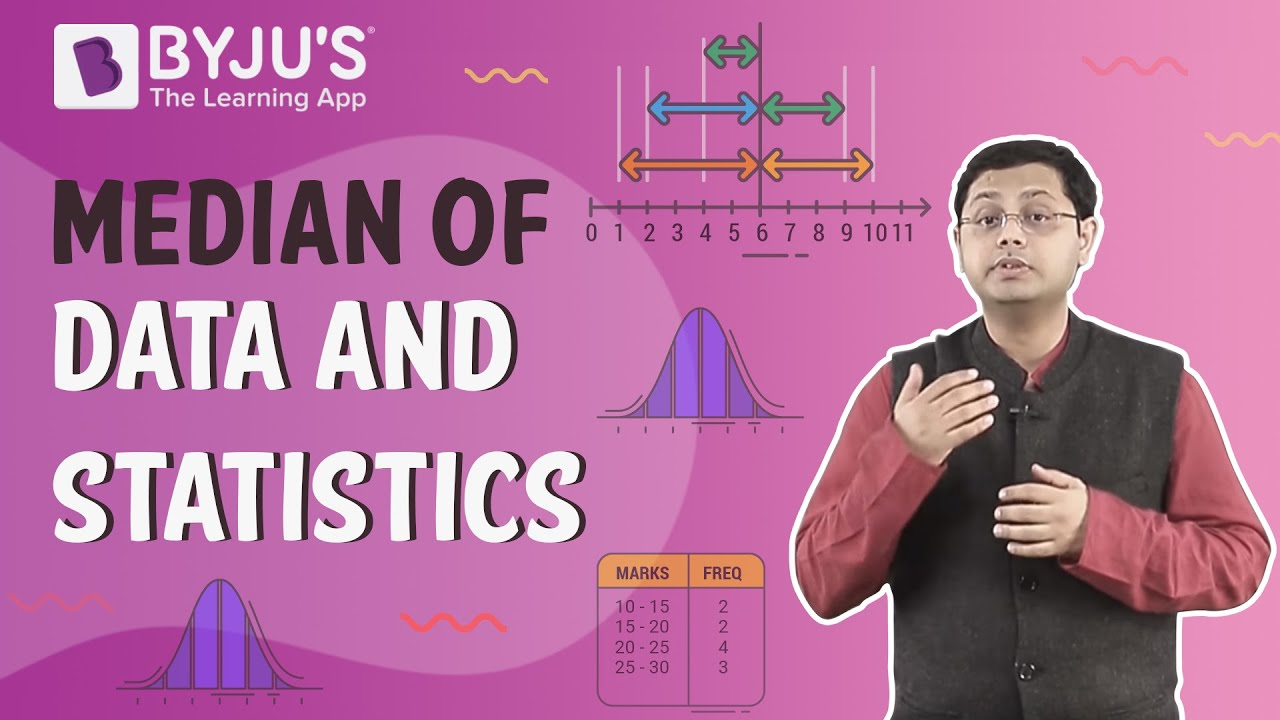# Calculate the median from the following data:

 Marks 0 – 10 10 – 30 30 – 60 60 – 80 80 – 90 Number of students 5 15 30 8 2

First, prepare the cumulative frequency table:

 Marks Number of students Cumulative frequency 0 – 10 5 5 10 – 30 15 5 + 15 = 20 30 – 60 30 20 + 30 = 50 60 – 80 8 50 + 8 = 58 80 – 90 2 58 + 2 = 60

Here N = 60

∴ n = 60/2 = 30

The cumulative frequency (c.f) just greater than 30 is 50.

The corresponding class (Median class) = 30 – 60

• l = 30
• c.f = 30
• F= 20
• h = 30
$$\begin{array}{l}Median = l + \frac{\frac{N}{2} – F}{c.f}\times h\end{array}$$
.

$$\begin{array}{l}Median = 30 + \frac{ 30 – 20}{30}\times 30\end{array}$$
.

Median = 40

For further information, check out the video### Refer more(38)(15)